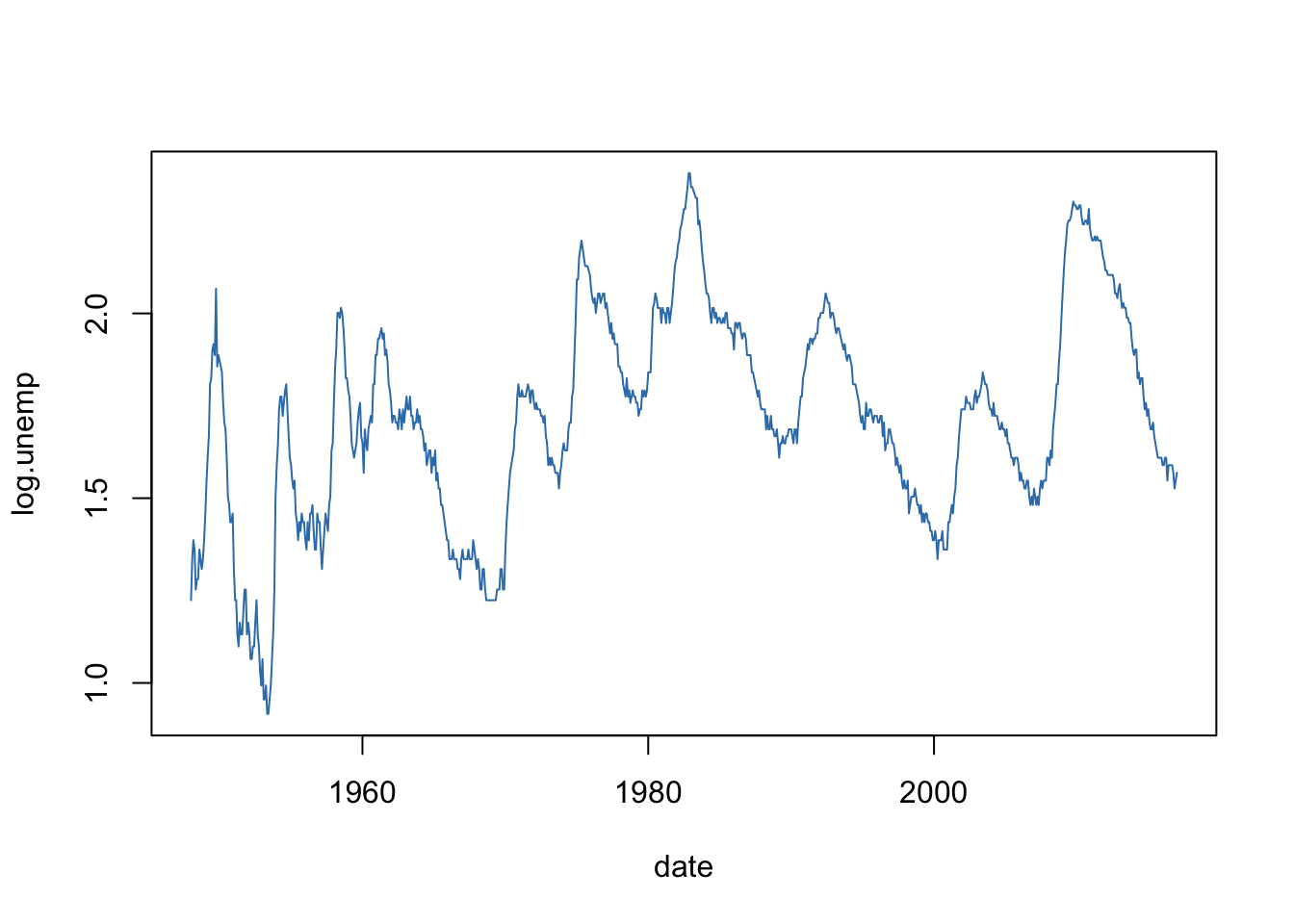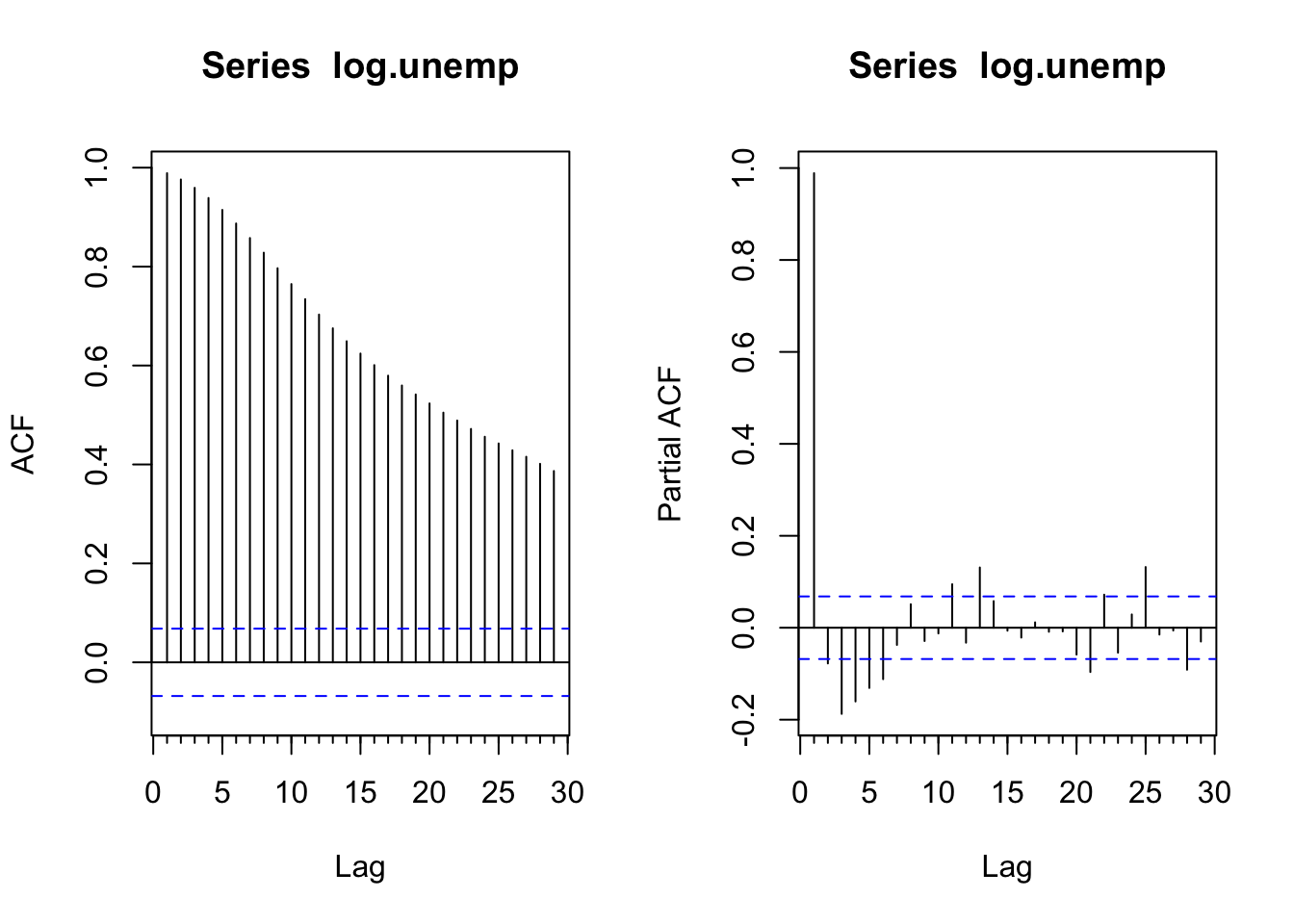``library("forecast")``

The dataset is the log unemployment rate, monthly, seasonally adjusted.

``````data <- read.csv("http://ptrckprry.com/course/forecasting/data/unemployment.csv")
date <- as.Date(data\$date)
time <- 1:length(date)
unemp <- data\$unemployment
log.unemp <- log(unemp)``````

Time series plot:

``plot(date, log.unemp, type="l", col=2)``ACF and PACF:

``````par(mfrow=c(1,2))
Acf(log.unemp)
Pacf(log.unemp)``````In HW5, we fit an ARIMA(2,1,2) model without a constant. The ACF of the residuals shows a clear seasonal correlation pattern (even though the original series was supposed to be seasonally adjusted).

``````fit1 <- Arima(log.unemp, c(2,1,2))
resid1 <- residuals(fit1)
Acf(resid1)``````